# Introduction

Consider the equilibrium configuration of an inhomogeneous self-gravitating celestial body, composed of incompressible fluid, that is rotating steadily about some fixed axis passing through its center of mass. The analysis of Section 6.5 implies that the body's equation of hydrostatic equilibrium (in the co-rotating frame) can be written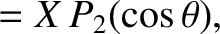(D.1)

where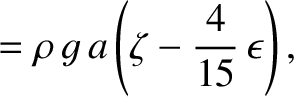(D.2)

and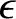(D.3)

Here,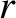,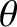,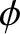are spherical coordinates whose symmetry axis coincides with the axis of rotation. Moreover,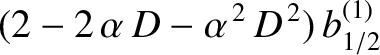is the fluid pressure,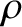the mass density,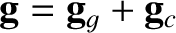the gravitational potential,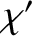the centrifugal potential, and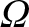the angular rotation velocity. Taking the curl of Equation (D.1), we deduce that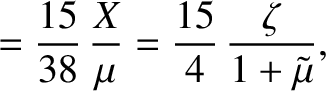, and, hence, that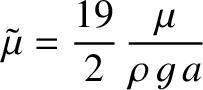(D.4)

Let us assume that the contours ofare spheroidal, so that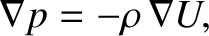(D.5)

where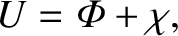(D.6)

Here,and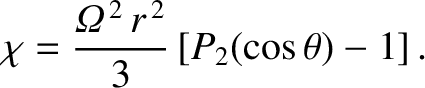are the mean radius and ellipticity of the density contour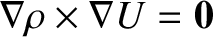, respectively. Furthermore, it is assumed that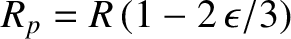. (See Section 3.6). It follows from Equations (D.4) and (D.5) that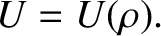(D.7)

The aim of this appendix is to deduce the relationship between the degree of flattening of the body, its rotational angular velocity, and its moment of inertia about its rotation axis.